# RD Sharma Class 8 Solutions Chapter 6 Algebraic Expressions and Identities Ex 6.4

In this chapter, we provide RD Sharma Class 8 Solutions Chapter 6 Algebraic Expressions and Identities Ex 6.4 for English medium students, Which will very helpful for every student in their exams. Students can download the latest RD Sharma Class 8 Solutions Chapter 6 Algebraic Expressions and Identities Ex 6.4 Maths pdf, free RD Sharma Class 8 Solutions Chapter 6 Algebraic Expressions and Identities Ex 6.4 Maths book pdf download. Now you will get step by step solution to each question.

### RD Sharma Solutions for Class 8 Chapter 6 Algebraic Expressions and Identities Ex 6.4Download PDF

Find the following products

Question 1.
2a3 (3a + 5b)

Solution:
2a3 (3a + 5b) = 2a3 x 3a + 2a3 x 5b
= 6a3 +1 + 10a3b
= 6a4 + 10a3b

Question 2.
-11a (3a + 2b)
Solution:
-11a (3a + 2b) = -11a x 3a – 11a x 2b
= -33a2– 22ab

Question 3.
-5a (7a – 2b)

Solution:
-5a (7a – 2b) = -5a x 7a- 5a x (-2b)
= -35a2 + 10ab

Question 4.
-11y2 (3y + 7)
Solution:
-11y2 (3y + 7) = -11y2 x 3y – 11y2 x 7
= -33y2+1-77y2
= 33y3-77y2

Question 5.
6×5 (x3+y3)

Solution: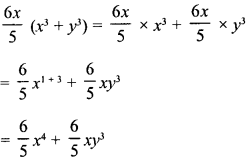Question 6.
xy (x3-y3)
Solution:
xy (x3 – y3) =xy x x3 – xy x y3
= x1 + 3 x y – x x y1+3
= x4y – xy4

Question 7.
0.1y (0.1x5 + 0.1y)
Solution:
0.1y (0.1x5 + 0.1y) = 0.1y x 0.1x5 + 0.1y x 0.1y
= 0.01x5y + 0.01y2

Question 8.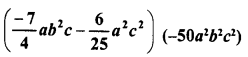Solution:Question 9.Solution:Question 10.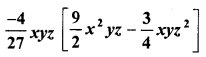Solution: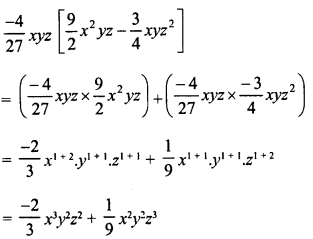Question 11.
5x (10x2y – 100xy2)
Solution:
5x (10x2y – 100xy2)
= 1.5x x 10x2y – 1.5x x 100xy2
= 15x1 + 2y- 150x1+1 x y2
15 x3y- 150xy2

Question 12.
4.1xy (1.1x-y)
Solution:
4.1xy (1.1x-y) = 4.1xy x 1.1x – 4.1xy x y
= 4.51x2y-4.1xy2

Question 13.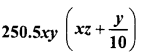Solution: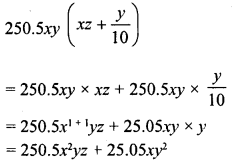Question 14.Solution:Question 15.
43 a (a2 + 62 – 3c2)
Solution: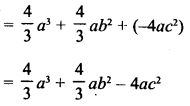Question 16.
Find the product 24x2 (1 – 2x) and evaluate its value for x = 3.

Solution:
24x2 (1 – 2x) = 24x2 x 1 + 24x2 x (-2x)
= 24x2 + (-48x2+1)
= 24x2 – 48x3
If x = 3, then
= 24 (3)2 – 48 (3)3
= 24 x 9-48 x 27 = 216- 1296
= -1080

Question 17.
Find the product of -3y (xy +y2) and find its value for x = 4, and y = 5.
Solution:
-3y (xy + y2) = -3y x xy – 3y x y2
= -3xy2 -3y2 +1  = -3xy2 – 3y3
If x = 4, y = 5, then
= -3 x 4 (5)2 – 3 (5)3 = -12 x 25 – 3 x 125
= -300 – 375 = – 675

Question 18.
Multiply – 32 x2y3 by (2x-y) and verify the answer for x = 1 and y = 2.

Solution:Question 19.
Multiply the monomial by the binomial and find the value of each for x = -1, y = 25 and z =05 :

(i) 15y2 (2 – 3x)
(ii) -3x (y2 + z2)
(iii) z2 (x – y)
(iv) xz (x2 + y2)
Solution: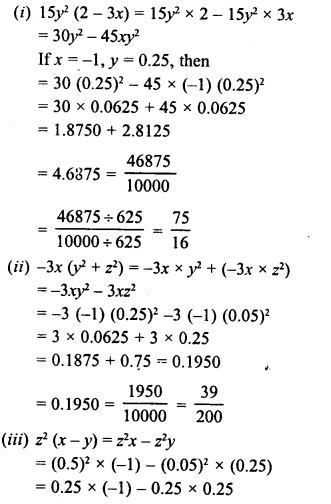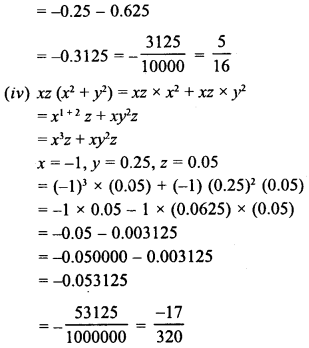Question 20.
Simplify :

(i) 2x2 (at1 – x) – 3x (x4 + 2x) -2 (x4 – 3x2)
(ii) x3y (x2 – 2x) + 2xy (x3 – x4)
(iii) 3a2 + 2 (a + 2) – 3a (2a + 1)
(iv) x (x + 4) + 3x (2x2 – 1) + 4x2 + 4
(v) a (b-c) – b (c – a) – c (a – b)
(vi) a (b – c) + b (c – a) + c (a – b)
(vii) 4ab (a – b) – 6a2 (b – b2) -3b2 (2a2 – a) + 2ab (b-a)
(viii) x2 (x2 + 1) – x3 (x + 1) – x (x3 – x)
(ix) 2a2 + 3a (1 – 2a3) + a (a + 1)
(x) a2 (2a – 1) + 3a + a3 – 8
(xi) 32-x2 (x2 – 1) + 14-x2 (x2 + x) – 34x (x3 – 1)
(xii) a2b (a – b2) + ab2 (4ab – 2a2) – a3b (1 – 2b)
(xiii )a2b (a3 – a + 1) – ab (a4 – 2a2 + 2a) – b (a3– a2 -1)
Solution:
(i)
2x2 (x3 -x) – 3x (x4 + 2x) -2 (x4 – 3x2)
= 2xx x3-2x2x x-3x x x4-3x x 2x-2x+ 6x2
= 2x2 + 3– 2x2 +1 – 3x,1+ 4-6x,1+1 -2x4 + 6x2
= 2x5 – 2x3 – 3x5 — 6x2 – 2x4 + 6x2
= 2x5 – 3x5 – 2a4 – 2x3 + 6x2 – 6x2
= -x5 – 2x4 – 2x3 + 0
= -x5-2x4-2x3
(ii) x3y (x2 – 2x) + 2xy (x3 – x4)
= x3y x x2 – x3y x 2x + 2ay x ac3 – 2xy x x4
= x3 + 2y-2x3 + 1 y + 2x1 + 3y – 2yx4+1
= x5y – 2x4y + 2x4y – 2yx5
= -x5y
(iii) 3a2 + 2 (a + 2) – 3a (2a + 1)
= 3a2 + 2a + 4 – 6a2 – 3a
= 3a2 – 6a2 + 2a – 3a + 4
= -3a2 – a + 4
(iv) x (x + 4) + 3x (2x2 – 1) + 4x2 + 4
= x2 + 4x + 3x x 2x2 – 3x x 1 + 4x2 + 4
= x2 + 4x + 6x2 +1 – 3x + 4x2 + 4
= x2 + 4x + 6x3 – 3x + 4x2 + 4
= 6a3 + 4x2 + x2 + 4x – 3x + 4
= 6x3 + 5x2 + x + 4
(v) a (b – c)-b (c – a) – c (a – b)
= ab – ac – be + ab – ac + bc
= 2ab – 2ac
(vi) a (b – c) + b (c – a) + c (a – b)
= ab – ac + bc – ab + ac – bc
= ab – ab + bc – be + ac – ac
= 0
(vii) 4ab (a – b) – 6a2 (b – b2) -3b2 (2a2 – a) + 2ab (b – a)
= 4a2b – 4ab2 – 6a2b + 6a2b2 – 6a2b2 + 3ab+ 2ab2 – 2a2b
= 4a2b- 6a2b – 2 a2b – 4ab2 + 3 ab2 + 2ab2 + 6a2b2 – 6a2b2
= 4a2b – 8a2b – 4ab2 + 5 ab2 + 0
= – 4a2b + ab2
(viii) x2 (x2 + 1) – x3 (x + 1) – x (x3 – x)
= x2 + 2 + x2 – x3 + 1 – x3 – x1 + 3 + x1 + 1
= x4 + x2-x4-x3-x4 + x2
= x4-x4-x4-x3 + x2 + x2
= -x4 – x3 + 2x2
(ix) 2a2 + 3a (1 – 2a3) + a (a + 1)
= 2a2 + 3 a – 3 a x 2a3 + a2 + a
= 2a2 + 3a – 6a1 + 3 + a2 + a
= 2a2 + 3a – 6a4 + a2 + a
= -6a4 + 3a2 + 4a
(x) a2 (2a – 1) + 3a + a3 – 8
= 2 a2 x a – a2 x 1+3a + a3-8
= 2a3 – a2 + 3a + a3 – 8
= 2a3 + a3 – a2 + 3a – 8
= 3a3 – a2 + 3a – 8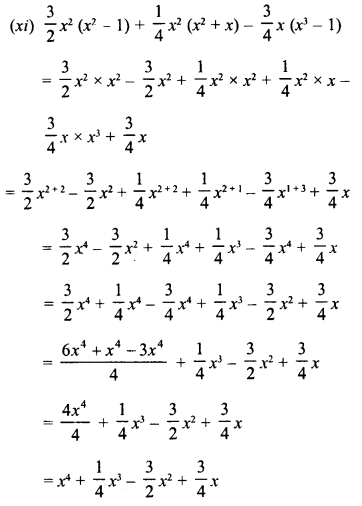(xii) a2b (a – b2) + ab2 (4ab – 2a2) – a3b (1 – 2b)
= a2b x a – a2b x b1 + ab2 x 4ab – ab1 x2a-a3b x 1 + a3b x 2b
= a2+1 b-a2b2 +1+ 4a1 +1 b2 +1 -2a2+1 b2-a3b + 2a3b1 +1
= a’b – a2b3 + 4a2b3 – 2a3b2 – a3b + 2a3b2
= a3b – a3b – a2b3 + 4a2b3 – 2a3b2 + 2a3b2
= 0 + 3a2b3 + 0 = 3 a2b3
(xiii) a2b (a3 – a + 1) – ab (a4 – 2a2 + 2a) – b (a-a2– 1)
= a2b x a3 – a2b x a + a2b – ab x a2 + ab x 2a2 – ab x 2a- ba3 + ba2 + b
= a2+ 3b – a2+1 b + a2b -a1 + 4b + 2a1 + 2b- 2a1+1 b- a3b + a2b + b
= a5b – a3b + a26 – a5b + 2a3b – 2a2b – a3b + a2b + b
= a5b – a3b + 2a3b – a36 – a3b + a2b – 2a2b + a2b + b
= a3b – a5b + 2a3b – 2a3b + 2a2b-2a2b + b
= 0 + 0 + 0 + b = b

All Chapter RD Sharma Solutions For Class 8 Maths

—————————————————————————–

All Subject NCERT Exemplar Problems Solutions For Class 8

All Subject NCERT Solutions For Class 8

*************************************************

I think you got complete solutions for this chapter. If You have any queries regarding this chapter, please comment on the below section our subject teacher will answer you. We tried our best to give complete solutions so you got good marks in your exam.

If these solutions have helped you, you can also share rdsharmasolutions.in to your friends.